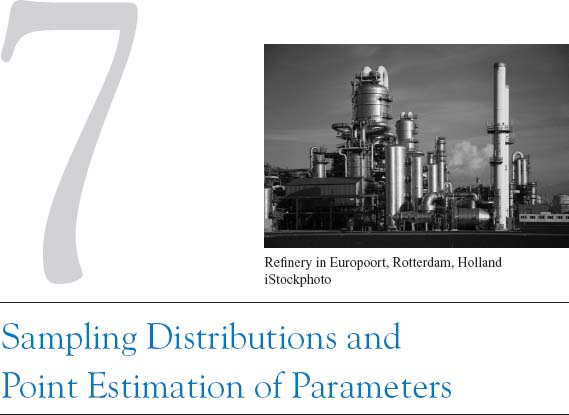## INTRODUCTION

Statistical methods are used to make decisions and draw conclusions about populations. This aspect of statistics is generally called statistical inference. These techniques utilize the information in a sample in drawing conclusions. This chapter begins our study of the statistical methods used in decision making.

Statistical inference may be divided into two major areas: parameter estimation and hypothesis testing. As an example of a parameter estimation problem, suppose that an engineer is analyzing the tensile strength of a component used in an automobile chassis. Variability is naturally present between the individual components because of differences in raw material batches, manufacturing processes, and measurement procedures (for example), so the engineer wants to estimate the mean strength of the population of components. In practice, the engineer will use sample data to compute a number that is in some sense a reasonable value (a good guess) of the true population mean. This number is called a point estimate. We will see that there are procedures for developing point estimates of parameters that have good statistical properties. We will also be able to establish the precision of the point estimate.

Now let’s consider a different type of question. Suppose that two different reaction temperatures t1 and t2 can be used in a chemical process. The engineer conjectures ...

Get Applied Statistics and Probability for Engineers, 5th Edition now with the O’Reilly learning platform.

O’Reilly members experience books, live events, courses curated by job role, and more from O’Reilly and nearly 200 top publishers.